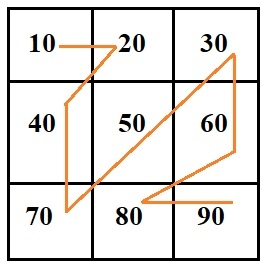# Print matrix in zag-zag fashion in C Programming.

Server Side ProgrammingProgrammingC

#### Beyond Basic Programming - Intermediate Python

Most Popular

36 Lectures 3 hours

#### C Programming from scratch- Master C Programming

Best Seller

60 Lectures 8 hours

#### C++ Programming

238 Lectures 56.5 hours

Given a matrix mat[row][col] we have to print the given matrix in zig-zag fashion like in the given image below −So the output should be like −

Output: 10 20 40 70 50 30 60 80 90

For the above problem, we have followed a simple approach where we have to iterate the matrix diagonally and change the value of iteration to change the direction after every previous match.

## Algorithm

START
STEP 1-> DECALRE AND SET k = 3, l = 3
STEP 2-> DECLARE A MATRIX mat[]
STEP 3-> DECLARE AND SET row = 0, col = 0, flag = false;
STEP 4-> SET mn = MINIMUM(k, l)
STEP 5-> LOOP FOR len = 1 AND len <= mn AND ++len
LOOP FOR i = 0 AND i < len AND ++i
PRINT mat[row][col]
IF i + 1 == len THEN,
BREAK
END IF
IF flag THEN,
INCREMENT row BY 1 AND DECREMENT col BY 1
ELSE
DECREMENT row BY 1 AND INCREMENT col BY 1
END IF
END FOR
IF len == mn THEN,
BREAK
END IF
IF flag THEN
INCREMENT row BY 1 AND SET flag = FALSE
ELSE
INCREMENT col BY 1 AND SET flag = TRUE
END IF
END FOR
STEP 6-> IF row == 0 THEN,
IF col == k – 1 THEN,
INCREMENT row BY 1
ELSE
INCREMENT col BY 1
END IF
SET flag = 1
ELSE
IF row == l – 1 THEN,
INCREMENT col BY 1
ELSE
INCREMENT row BY 1
SET flag = 0
END IF
STEP 7-> SET MAX = MAXIMUM(k, l) – 1
STEP 8-> LOOP FOR len, diag = MAX AND diag > 0 AND --diag
IF diag > mn THEN,
SET len = mn
ELSE
SET len = diag
END IF
FOR i = 0 AND i < len AND ++i
PRINT mat[row][col]
IF i + 1 == len THEN,
BREAK
END IF
IF flag THEN,
INCREMENT row BY 1 AND DECREMENT col BY 1
ELSE
INCREMENT col BY 1 AND DECREMENT row BY 1
END IF
IF row == 0 || col == k – 1 THEN,
IF col == k - 1
INCREMENT row BY 1
ELSE
INCREMENT col BY 1
END IF
SET flag = true
ELSE IF col == 0 || row == l – 1 THEN,
IF row == l – 1 THEN,
INCREMENT col BY 1
ELSE
INCREMENT row BY 1
END IF
SET flag = false
ENF IF
END FOR
STOP

## Example

Live Demo

#include <stdio.h>
#include <stdbool.h>
#define C 3
#define min(a, b) a>b?b:a
#define max(a, b) a>b?a:b
int main(){
int k = 3, l = 3;
int mat[] = {
{ 10, 20, 30 },
{ 40, 50, 60 },
{ 70, 80, 90 }
};
int row = 0, col = 0;
bool flag = false;
int i, j, len, diag;
int MAX;
int mn = min(k, l); //to check the minimum number and return that minimum number
for ( len = 1; len <= mn; ++len) {
for ( i = 0; i < len; ++i) {
printf("%d ", mat[row][col]); //Printing the matrix in zigzag format
if (i + 1 == len)
break;
if (flag)
++row, --col;
else
--row, ++col;
}
if (len == mn)
break;
if (flag)
++row, flag = false;
else
++col, flag = true;
}
if (row == 0) {
if (col == k - 1)
++row;
else
++col;
flag = 1;
} else {
if (row == l - 1)
++col;
else
++row;
flag = 0;
}
MAX = max(k, l) - 1; //To check the maximum element
for ( len, diag = MAX; diag > 0; --diag) { //Loop to go diagonally.
if (diag > mn)
len = mn;
else
len = diag;
for ( i = 0; i < len; ++i) {
printf("%d ", mat[row][col]);
if (i + 1 == len)
break;
if (flag)
++row, --col;
else
++col, --row;
}
if (row == 0 || col == k - 1) {
if (col == k - 1)
++row;
else
++col;
flag = true;
}
else if (col == 0 || row == l - 1) {
if (row == l - 1)
++col;
else
++row;
flag = false;
}
}
return 0;
}

## Output

This Program will Print output −

10 20 40 70 50 30 60 80 90